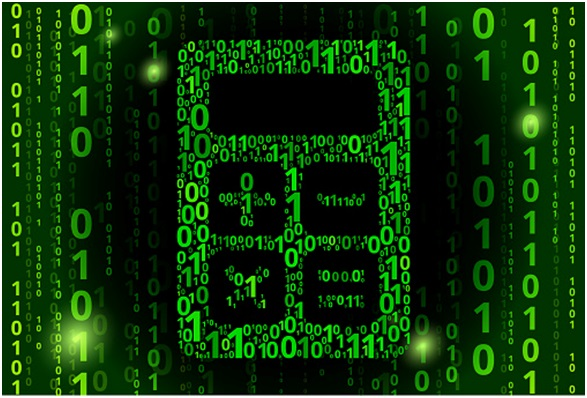Url.https'>

### Convert Numbers To Binary, Hexadecimal, Decimal, Octal Number SystemThe service is designed to convert numbers from one number system to another in online mode.
To do this, select the base of the system from which you want to convert the number. You can enter both integers and semicolons. Online binary calculator for work with binary arithmetic. The number of units in the binary notation of the decimal number.

### Ways to represent numbers

Binary numbers - each digit means the value of one bit (0 or 1), the most significant bit is always written to the left, the letter “b” is put after the number. For ease of perception, tetrads can be separated by spaces. For example, 1010 0101b.
Hexadecimal numbers - each tetrad is represented by a single character 0 ... 9, A, B, ..., F. Such a representation can be designated differently, only the “h” symbol after the last hexadecimal digit is used here. For example, A5h. In the texts of programs, the same number can be designated as 0xA5 and 0A5h, depending on the syntax of the programming language. A non-significant zero (0) is added to the left of the most significant hexadecimal digit represented by the letter to distinguish between numbers and symbolic names.
Decimal(decimal) numbers - each byte (word, double word) is represented as a normal number, and the decimal notation (letter “d”) is usually omitted. The byte from the previous examples has a decimal value of 165. Unlike the binary and hexadecimal notation, it is difficult to determine the value of each bit in decimal to binary, which sometimes has to be done.
Octal numbers - each three bits (the division begins with the lowest) is written in the form of the number 0–7, at the end the sign “o” is set. The same number will be written as 245 °. The octal system is inconvenient in that the byte cannot be divided equally.

### Description of the online calculator

• The maximum size of the entered numbers is 16 characters
• You can enter only integer and negative numbers in the value input fields
• From 11 to 16 of the number systems, the following designations are used during input: A-10, B-11, C-12, D-13, E-14, F-15
• In the input fields of the base of the numeration system, you can enter only a positive number from 2 to 16.

Algorithm for translating numbers from one number system to another
The conversion of integer decimal numbers to any other number system is carried out by dividing the number by the base of the new number system until there is no residue less than the base of the new number system. The new number is written as the remainder of the division, starting with the last.
The transfer of the correct decimal fraction to another MSS is carried out by multiplying only the fractional part of the number by the base of the new number system until all the zeros remain in the fractional part or until the specified translation accuracy is achieved. As a result of each multiplication operation, one digit of a new number is formed starting with the oldest.
The translation of the wrong fraction is carried out according to rules 1 and 2. The integer and fractional parts are written together, separated by a comma.

Thanks a lot for reading along. What do you think? Kindly drop us a comment and share to your friends.
Previous
Next Post »